# How to Calculate Elapsed Time

Elapsed time and time duration formula, techniques, and practiceAuthor
Tess LouckaPublished
October 11, 2023# How to Calculate Elapsed Time

Elapsed time and time duration formula, techniques, and practiceAuthor
Tess LouckaPublished
Oct 11, 2023# How to Calculate Elapsed Time

Elapsed time and time duration formula, techniques, and practiceAuthor
Tess LouckaPublished
Oct 11, 2023Key takeaways

• Elapsed time is the time that exists between the start and end of an event
• Calculating elapsed time is one of the most common types of mathematics that we use in our daily lives
• The formula for elapsed time is Elapsed Time = End Time – Start Time

Picture this: it’s 3:15 and you’re at home getting ready for a birthday party. You have to leave at 5:45 but you still need to pick out your outfit and wrap your present!

How much time do you have to finish getting ready?

Calculating elapsed time is one of the most common forms of mathematics we use in our daily lives, but what is it?

## What is Elapsed Time?The word “elapse” means to pass by. When used to talk about time, it means the amount of time that has passed between the start and end of an event. E.g., the time between now (3:15) and when you have to leave to get to the birthday party on time (5:45).

Said another way, elapsed time is  the difference between two times.

Elapsed time is sometimes called time duration. Duration is the amount of time it takes for something to take place or how long an event continues. To calculate duration time would be the same as calculating elapsed time

## Real-World Examples of Elapsed TimeHow often do you look at the clock to figure out how many hours are left until school is over, how many episodes of your favorite show you can watch until dinner, or how much time you have to study before a test?

You use elapsed time in each of those examples. The ability to calculate time duration also comes in handy when figuring out how long it will take to follow a recipe, finish a movie, or drive to school.

Elapsed time can be used to calculate the time between dates on a calendar, too.

When calculating elapsed time in terms of days or months, knowing the number of days in each month of the year, including leap years, will come in handy!

## How to Calculate Elapsed TimeBefore you can calculate elapsed time, you must know how to tell time and how to subtract. These are the foundation of time duration. Once you have a strong foundation, you can use the following techniques for calculating elapsed time: the elapsed time formula, the “counting-up” technique or a number line.

### Formula to Calculate Time DurationUsing the elapsed time formula below, let’s revisit our birthday party example. If it’s 3:15 PM and the party starts at 5:45 PM, how much time do you have to get ready for the birthday party?.

Elapsed Time = End Time – Start Time

To solve the problem, we need to subtract the start time from the end time. By plugging our start and end time into the equation we can solve for elapsed time.

5:45 – 3:15 = 2 hours and 30 minutes

This means there are 2 hours and 30 minutes between 3:15 and 5:45. So, you have 2 hours and 30 minutes to finish getting ready before you have to leave for the birthday party!

### The 'Counting-Up' TechniqueAnother technique that makes calculating time duration easier is the “counting-up” technique. Count up from the starting time by hours. Once you get to the same hour as the end time, either add or subtract the start time’s minutes to equal the end time’s minutes.

Take 6:20 PM and 9:00 PM, for example. Count up from the start time (6:20) by hours until you reach the end time (9:00) — 7:20, 8:20, 9:20. That’s 3 hours. However, 9:20 is 20 minutes past our end time of 9:00. Therefore, we need to subtract 20 minutes from 3 hours to get our answer.

3 hours – 20 minutes = 2 hours and 40 minutes. That’s the elapsed time from 6:20 to 9:00.

### Calculate Duration of Time on a Number LineTo calculate the duration of time, you can draw a line with the start time at one end and the end time at the other.

If the start time is not directly on the hour, add up the minutes to the next greatest hour. Do the same with the end time but to the next smallest hour. Add up the hours in between. Then, add the minutes.

Let’s try this out using the times 3:48 and 8:19.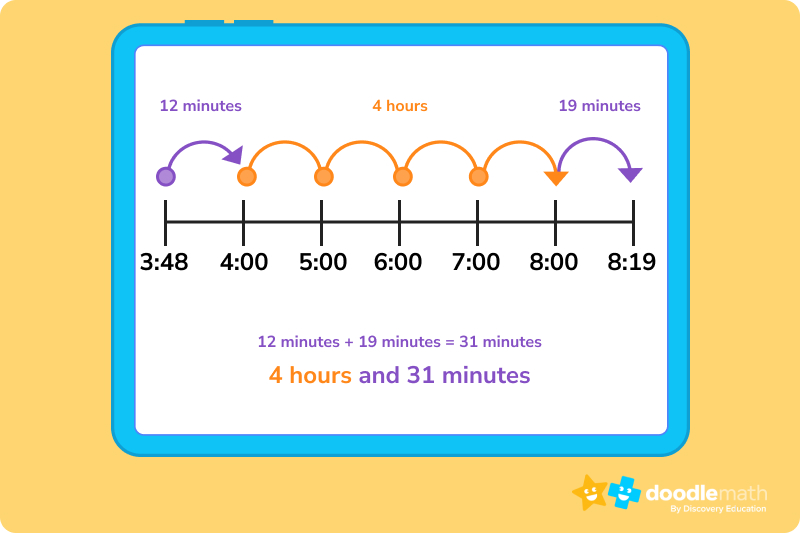The start time of 3:48 is not directly on an hour, so we’ll add up the minutes to the next greatest hour, which is 4:00.

3:48 + 12 minutes = 4:00

The end time of 8:19 is also not directly on an hour, so we’ll subtract the minutes to the previous hour, which is 8:00.

8:19 – 19 minutes = 8:00.

To get from 4:00 to 8:00, we count 4 hours on the number line. Next, we add the additional minutes we calculated together.

12 + 19 = 31

Finally, we add the hours to the minutes to get the total elapsed time.

4 hours + 31 minutes = 4 hours and 31 minutes

So, the elapsed time between 3:48 and 8:19 is 4 hours and 31 minutes.

For more practice on calculating time duration, pay attention to the times things take place in your day-to-day life. Figure out how long your classes are, how long a show is, or how much time there is between when you go to bed and when you wake up.

For additional support with clock reading and time calculations, you can use a math website or math app

## Practice problems

Answer: 3 hours and 45 minutes

Answer: 5 hours and 49 minutes

Answer: 1 hour and 5 minutes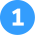Question 1

Use the counting-up technique. From 11 a.m. to 2:00 p.m. is 3 hours. Add the 45 minutes to that to get 3 hours and 45 minutes. You can use the elapsed time formula or a number line to solve this problem too. Make sure your child knows the difference between AM and PM when calculating the elapsed time.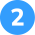Question 2

Use the number line technique. Calculate the minutes between 3:19 and the next greatest hour (4:00) to get 41 minutes. Calculate the minutes between 9:08 and the previous hour (9:00) to get 8 minutes. To get from 4:00 to 9:00, you count 5 hours. Next, add your minutes together (41 + 8 = 49) and then add 49 minutes to 5 hours to get the final answer of 5 hours and 49 minutes.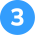Question 3

Plug the start time and end time into the elapsed time formula to solve for elapsed time.

6:20 – 5:15 = 1 hour and 5 minutes.Elapsed time is the amount of time that has passed between the start and end of an event or given time frame.

There are 3 hours in between 2:00 p.m. and 5:00 p.m. Three hours is an example of elapsed time.

You can count elapsed time using the elapsed time formula, a number line, or the counting-up technique.Lesson credits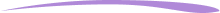Tess Loucka

Tess Loucka discovered her passion for writing in high school and has not stopped writing since. Combined with her love of numbers, she became a math and English tutor, focusing on middle- and high-school-level topics. Since graduating from Hunter College, her goal has been to use her writing to spread knowledge and the joy of learning to readers of all ages.

Tess Loucka

Tess Loucka discovered her passion for writing in high school and has not stopped writing since. Combined with her love of numbers, she became a math and English tutor, focusing on middle- and high-school-level topics. Since graduating from Hunter College, her goal has been to use her writing to spread knowledge and the joy of learning to readers of all ages.# Are you a parent, teacher or student?

Are you a parent or teacher?

## Hi there!

Book a chat with our team